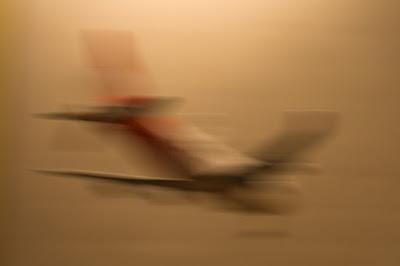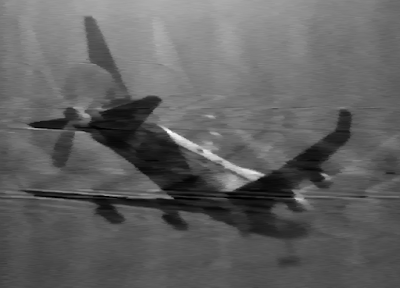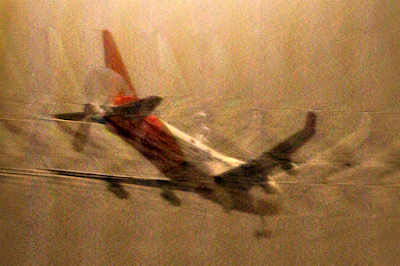## Wednesday, November 18, 2015

### Image deblur experiments

Quite some time ago a friend of mine took a photo, which turned out heavily motion blurred. I had just been talking about image deblurring so he sent the photo to me to see what I could do with it. I spent some time working on the photo and computed a reconstruction, which was quite good.Figure 1. The original photo as I received it.Figure 2. My original reconstruction from some years ago.

The original photo is shown in Figure 1, while my original reconstruction is shown in Figure 2. In Figure 1 one can see that the object appears to be an airplane. Figure 2 however reveals that the object in question is in fact an airplane toy, which is apparent from the propeller in the rear. I was genuinely surprised when I saw this in the reconstruction as I believed the photo was of a real plane and the reconstruction had actually provided new information.

My original reconstruction relied on the fact that the convolution kernel is essentially one dimensional, and it was in grayscale due to computational limitations. This time I wanted to try to reconstruct the image using a more generic approach and in color.

In the original reconstruction I used a total variation regularized deconvolution applied to each horizontal line of the image separately. This could be done because the blur happens almost exactly in the horizontal direction (in fact I rotated the photo slightly to make this even more the case). The convolution kernel was selected by analyzing the photo by eye and computing several simple Tikhonov regularized reconstructions with different kernels and choosing the one which looked the best.

This time around I wanted to use a 2D total variation regularized deconvolution using the trick of writing the problem as a constrained quadratic programming problem. Some poorly formatted maths follow:

The problem itself is

min_X 1/2 || conv(K,X) - B ||^2 + alpha sum {|X_(i+1,j)-X_(i,j)|+|X_(i,j+1)-X_(i,j)|},

where conv(K,X) is the convolution of image X with kernel K, || || denotes the 2-norm while | | denotes the absolute value. The values |X_(i+1,j)-X(i,j)| and |X(i,j+1)-X(i,j)| are the variations in the image and summing up all of them over the image is the total variation, hence the name total variation regularization. The target function is not differentiable and thus this is quite a difficult optimization problem. The trick however is to notice that this can be re-written as

min_X 1/2 || conv(K,X) - B ||^2 + alpha sum { U_k + V_k },

with the constraints that LX = U-V and U,V>=0, where L is an operator which gives the (signed) variations X_(i+1,j)-X_(i,j) and X_(i,j+1)-X_(i,j) for all i,j. U then represents the positive part of the variations of X while V represents the negative part. The total variation is then just the sum of the components of U and V.

There are multiple ways to actually find the minimizer of the latter formulation, of which I've implemented the projected Barzilai-Borwein method due to its simplicity. I enforce the equality constraint with a penalty term to keep the system positive-definite (although I'm currently working on doing this with some sort of stabilized Uzawa iteration to solve the saddle point problem one arrives at when enforcing the constraint using a Lagrange multiplier). The Hessian of the system is not only large (but not huge: about 5 million unknowns), but also dense (around 10 billion nonzeros), which makes iterative methods the only possible option. Fast Fourier transforms save the day.

As was the case with the earlier reconstruction, I again started with analyzing the photo by eye, choosing a suitable type of convolution kernel and narrowing the parameters of the kernel down. This was again done by computing several Tikhonov regularized reconstructions and choosing the one which looked the best.Figure 3. Tikhonov regularized deconvolution with alpha = 0.001 and with the most eye-pleasing convolution kernel.

Obviously I chose the kernel type as a moving average over a straight line with the parameters being the length of the line and its angle from horizontal. The values of the parameters found to give best looking results were length = 110 pixels and angle = 0.03 radians. Figure 3 shows the result when using a standard Tikhonov regularization with regularization parameter alpha = 0.001. This computation takes 5 seconds on my 9 year old laptop.Figure 4. Total variation regularized deconvolution with alpha = 0.0005.

The result of the total variation regularized deconvolution with regularization parameter alpha = 0.0005 is shown in Figure 4. This computation takes several hours on my laptop, but I'm expecting this to reduce by quite a lot by using more elaborate algorithms.Figure 5. Total variation regularized deconvolution (alpha = 0.0005) using a kernel estimated from the reconstruction in Figure 4.

As the total variation regularization reduces the ghosting artifacts (which I'm guessing are due to the kernel not being quite right), I was wondering if I could use the reconstruction to give a better estimate of the convolution kernel. As the kernel is just the deconvolution of the blurred image using the sharp image, I could quickly compute the kernel using a Tikhonov regularized deconvolution. When I then re-ran the deconvolution (again alpha = 0.0005) using this new kernel estimate, I got what is shown in Figure 5, which to my eye looks like the best one yet.